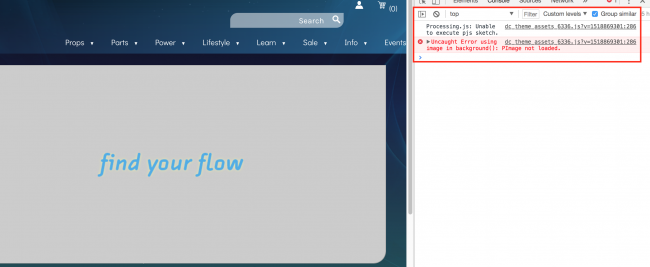#### Howdy, Stranger!

We are about to switch to a new forum software. Until then we have removed the registration on this forum.

# Unable to Get Background Image Working Online

edited February 2018

Hey guys. I have added a background image to my code which shows fine when I run this in Processing 3.3.6, however, when I host this on my website I get the following error in the console log before the background goes grey:`dc_theme_assets_6336.js?v=1518869301:286 Uncaught Error using image in background(): PImage not loaded.`

I don't really understand why this would be any different. I don't have much luck when searching online for this particular error in relation to my issue, so I'm wondering if anyone could share any insight?

Please see my full code below. The background image 'bannerbg.jpg' on line 20. Thanks so much.

``````Particle p = new Particle();

final int LIGHT_FORCE_RATIO = 70;
final int  LIGHT_DISTANCE= 70 * 50;
final int  BORDER = 100;
float baseRed, baseGreen, baseBlue;
float baseRedAdd, baseGreenAdd, baseBlueAdd;
final float RED_ADD = 3.2;
final float GREEN_ADD = 1.7;
final float BLUE_ADD = 4.3;
float boxX, boxY;
float widthSize = width;

int rectWidth = 915;
int rectHeight = 197;

PImage img;

void setup() {
img = loadImage("https://"+"s3.amazonaws.com/zcom-media/sites/a0iE000000QK9yZIAT/media/mediamanager/bannerbg.jpg");

background(img);
size(1840, 400);
// surface.setResizable(true);
noCursor();
//img = loadImage("flowtoy.jpg");
baseRed = 0;
baseRedAdd = RED_ADD;

baseGreen = 0;
baseGreenAdd = GREEN_ADD;

baseBlue = 0;
baseBlueAdd = BLUE_ADD;

boxX = width/2;
boxY = height/2;

}

void draw() {
//image(img, 400, 100, img.width/2, img.height/2);
if (mouseX>boxX-rectWidth && mouseX<boxX+rectWidth &&
mouseY>boxY-rectHeight && mouseY<boxY+rectHeight) {

//saveFrame("output/LightDrawing_####.png");

baseRed += baseRedAdd;
baseGreen += baseGreenAdd;
baseBlue += baseBlueAdd;

colorOutCheck();

p.move(mouseX, mouseY);

int tRed = (int)baseRed;
int tGreen = (int)baseGreen;
int tBlue = (int)baseBlue;

tRed *= tRed;
tGreen *= tGreen;
tBlue *= tBlue;

loadPixels();

int left = max(0, p.x - BORDER);
int right = min(width, p.x + BORDER);
int top = max(0, p.y - BORDER);
int bottom = min(height, p.y + BORDER);

for (int y = top; y < bottom; y++) {
for (int x = left; x < right; x++) {
int pixelIndex = x + y * width;

int r = pixels[pixelIndex] >>16 & 0xFF;
int g = pixels[pixelIndex] >> 8 & 0xFF;
int b = pixels[pixelIndex] & 0xFF;

int dx = x - p.x;
int dy = y - p.y;
int distance = (dx *dx ) + (dy* dy);

if (distance < LIGHT_DISTANCE) {
int fixFistance = distance * LIGHT_FORCE_RATIO;

if (fixFistance == 0) {
fixFistance = 1;
}
r = r + tRed / fixFistance;
g = g + tGreen / fixFistance;
b = b + tBlue / fixFistance;
}
pixels[x + y * width] = color(r, g, b);
}
}

updatePixels();
} else {
setup();
}

//updatePixels();
}

void colorOutCheck() {
if (baseRed < 10) {
baseRed = 10;
baseRedAdd *= -1;
} else if (baseRed > 255) {
baseRed = 255;
baseRedAdd *= -1;
}

if (baseGreen < 10) {
baseGreen = 10;
baseGreenAdd *= -1;
} else if (baseGreen > 255) {
baseGreen = 255;
baseGreenAdd *= -1;
}

if (baseBlue < 10) {
baseBlue = 10;
baseBlueAdd *= -1;
} else if (baseBlue > 255) {
baseBlue = 255;
baseBlueAdd *= -1;
}
}

void mousePressed()
{
setup();
}

class Particle {
int x, y;
float vx, vy;
//float slowLevel;

Particle() {
x = (int)3;
y = (int)2;
//   slowLevel = random(100) + 1;
}

void move(float targetX, float targetY) {
vx = (targetX - x) ;
vy = (targetY - y) ;

x = (int)(x + vx);
y = (int)(y + vy);
}
}
``````
Tagged:

## Answers

• ``````void mousePressed() {
setup();
}
``````

Wha?

• koogs I think this is to reset the animation when it's clicked on. Would that make sense? Unfortunately I'm picking this code up from an ex-employee so I'm not 100% on everything.

• GoToLoop, taking a look now - thank you

• Hi, About your code, I have some questions. tRed *= tRed; tGreen *= tGreen; tBlue *= tBlue; I don't understand what square is for =_= And is the following code to define the changing area of the pixel?

``````int left = max(0, p.x - BORDER);
int right = min(width, p.x + BORDER);
int top = max(0, p.y - BORDER);
int bottom = min(height, p.y + BORDER);
``````
• Hi LYY. Sorry, I'm not sure, this code was inherited by our company.

Sign In or Register to comment.